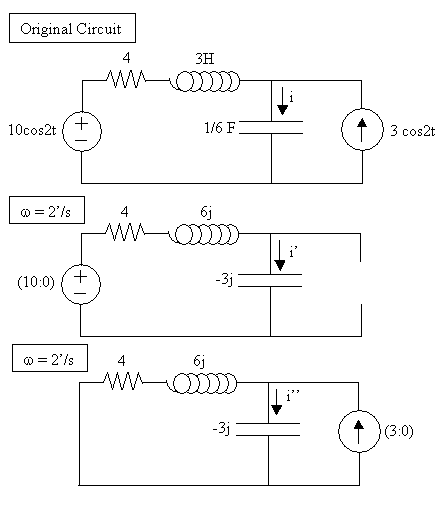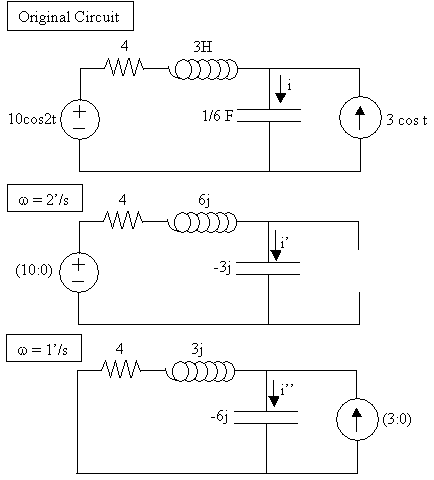More Analysis Techniques using Phasors
Nodal, Mesh, and Superposition Analysis

 Outline: Nodal Analysis Mesh Analysis When Superposition is necessary

Nodal Analysis

Any analysis techniques used for DC circuits can be applied for AC circuits.
This is true because all analysis techniques are based on Ohm's Law and Kirchoff's Laws.
These basic physical laws apply to ALL circuits.

Therefore we can apply Nodal Analysis to an AC circuit. All the steps are exactly the same as before,
the only difference is that phasors are used. Thus the math involves complex numbers.

The set-up is exactly the same for both DC and AC circuits.
Refer to the steps outlined in Lecure 5.

## Study Problems

After clicking on the following link enter 10-1 for the problem and 1 for the step:
Study Problem 10-1

Top of Page

Mesh Analysis

We can also apply Mesh Analysis to an AC circuit. All the steps are exactly the same as before,
the only difference is that phasors are used. Again the math involves complex numbers.

The set-up is exactly the same for both DC and AC circuits.
Refer to the steps outlined in Lecure 5.

## Study Problems

After clicking on the following link enter 10-2 for the problem and 1 for the step:
Study Problem 10-2

Top of Page

When Superposition is necessary.

Superposition is a technique where the circuit is analyzed with one source at a time.
In other words, if there are multiple sources in a circuit we can remove or 'kill' all sources
except for one and analyze the circuit. This is done for all sources (each one acting by itself
with all other sources 'killed'). When analysis is done for every source, then all the partial

If the ω's are all the same then the partial answers can be added to give one final answer.
However if the ω's are different, then the partial answers can not be combined.

In the following two AC problems we will use superposition to find
the current i through the capacitor.
 This circuit has two sources with the same ω: Since both sources have the same ω then superpostion is optional. In other words any other analysis technique could be used to find i, such as mesh or nodal analysis. Step 1: To use superpostion, we first 'kill' the current source. Killing a source means making the source zero. A current source with 0A is an OPEN CIRCUIT. To find i' we will simply use Ohm's Law: Zeq. = (4 + 6j -3j) = (4 + 3j) i' = (10:0)/(4 + 3j) (4 + 3j) = (5:37o) i' = (10:0)/(5:37o) = (2:-37o) Step 2: We will now kill the independent voltage source. OV means a SHORT CIRCUIT. To find i'' we will use Current Division: i'' = (3:0)[(4 + 6j)/(4 + 3j)] i'' = (3:0)[( 7.7  :56o)/(  5  :37o)] i'' = (3:0)[( 1.5  :19o)] i'' = ( 4.5 : 19o) Step 3: Now to find the original i: i = i' + i'' = ( 2 :-37o) + ( 4.5 : 19o) Since i' and i'' have the same ω we can add these two numbers: Converting i' to rectangular form: i'= 1.6 - 1.2j Converting i'' to rectangular form: i'' = 4.3 + 1.5j i = i' + i'' = (1.6 + 4.3) + (-1.2 + 1.5)j = 5.9 + 0.3j Now putting i back into the time domain: i = (6:2.9o) i = 6 cos (2t + 2.9o)This circuit has two sources with different ω's: Here it is mandatory to use superpostion because the ω's are different. If we didn't use superposition then we would not know which ω to use. Step 1:   ω=2 '/s First kill the current source: i' = 10/(4 + 6j - 3j) = 10/(4 + 3j) = 10/(5:37o) i' = (2:-37o) Step 2:   ω=1 '/s Now kill the voltage source: i'' = (3:0)[(4 + 3j)/(4 + 3j - 6j)] = (3:0)[(4 + 3j)/(4 - 3j)] i'' = (3:0)[(5:37o)/(5:-37o)] i'' = (3:0)(1:74o) i'' = (3:74o) Step 3: Now to find the original i: i = i' + i'' i' = 2cos(2t - 37o) i'' = 3cos(t + 74o) Since i' and i'' have different ω's, we can not add up the complex numbers to get one complex number for i. i = 2cos(2t - 37o) + 3cos(t + 74o)## Study Problems

After clicking on the following link enter 10-3 for the problem and 1 for the step:
Study Problem 10-3

Top of Page
Back To Index